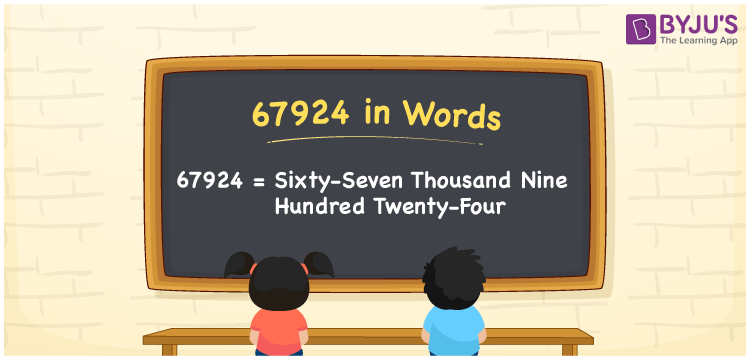# 67924 in Words

67924 in words is written as Sixty-seven thousand nine hundred twenty-four. In both the International System of Numerals and the Indian System of Numerals, 67924 is written as Sixty-seven thousand nine hundred twenty-four. The number 67924 is a Cardinal Number as it denotes some quantity. For example, “that motorcycle costs 67924 rupees”.

 67924 in Words Sixty-seven thousand nine hundred twenty-four Sixty-seven thousand nine hundred twenty-four in Number 67924

## 67924 in English Words

67924 in English words is read as “Sixty-seven thousand nine hundred twenty-four”.## How to Write 67924 in Words?

To write 67924 in words, we shall use the place value chart. In the place value chart, put 6 in the ten thousands, 7 in the thousands, 9 in the hundreds, 2 in the tens and 4 in the ones. Let us make a place value chart to write the number 67924 in words.

 Ten Thousands Thousands Hundreds Tens Ones 6 7 9 2 4

Thus, we can write the expanded form as

6 × Ten Thousand + 7 × Thousand + 9 × Hundred + 2 × Ten + 4 × One

= 6 × 10000 + 7 × 1000 + 9 × 100 + 2 × 10 + 4 × 1

= 60000 + 7000 + 900 + 20 + 4

= 67924

= Sixty-seven thousand nine hundred twenty-four.

67924 is a natural number, the successor of 67923 and the predecessor of 67925.

67924 in words – Sixty-seven thousand nine hundred twenty-four

• Is 67924 an odd number? – No
• Is 67924 an even number? – Yes
• Is 67924 a perfect square number? – No
• Is 67924 a perfect cube number? – No
• Is 67924 a prime number? – No
• Is 67924 a composite number? – Yes

## Frequently Asked Questions on 67924 in Words

Q1

### How to write 67924 in words?

67924 in words is written as Sixty-seven thousand nine hundred twenty-four.
Q2

### How to write 67924 in the International and Indian System of Numerals?

In both, the system of numerals, 67924 in words, is written as Sixty-seven thousand nine hundred twenty-four.
Q3

### How to write 67924 in a place value chart?

In the place value chart, write 6 in the ten thousands, 7 in the thousands, 9 in the hundreds, 2 in the tens and 4 in the ones, respectively.